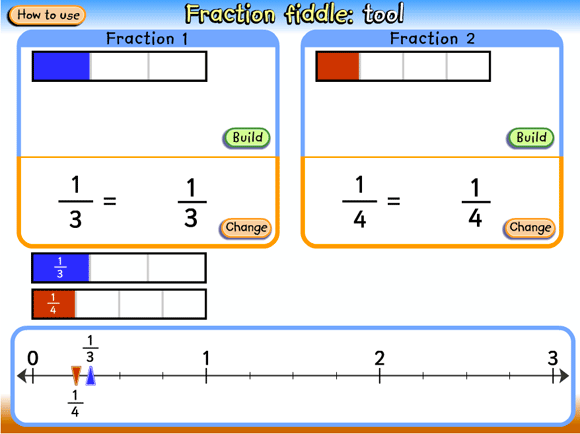Home > Fractions > Activities > Fraction fiddle

# Fraction fiddle

Display the learning object L2800 Fraction fiddle: tool to the class on an interactive whiteboard (or projector and screen).Screen grab from L2800 Fraction fiddle: tool.
Source: © Education Services Australia Ltd, 2011

• Enter a fraction (e.g. $$\frac{1}{3}$$) and discuss how a bar can be constructed to represent that fraction.
• Point out that the length of the bar is the same as the distance between 0 and 1 on the number line, and so helps to locate the fraction on the number line. The fraction bar acts as a bridge from the typical area model for representing fractions to a linear model.
• Select another fraction (e.g. $$\frac{1}{4}$$) and ask students to predict what the bar diagram will look like, and where the fraction will be located on the number line. Ask questions such as:
• Will $$\frac{1}{4}$$be smaller or larger than $$\frac{1}{3}$$?
• Will $$\frac{1}{4}$$ be closer to 1 than $$\frac{1}{3}$$?
• Emphasise the fact that, together, the numerator and denominator form a number that has a value, and therefore a place on the number line.